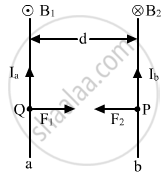Share

# Two Long Straight Parallel Conductors 'A' and 'B', Carrying Steady Currents Ia And Ib Are Separated by a Distance D. Write the Magnitude and Direction of the Magnetic Field Produced by the Conductor - Physics

ConceptForce Between Two Parallel Currents, the Ampere

#### Question

Two long straight parallel conductors 'a' and 'b', carrying steady currents Ia and Ib are separated by a distance d. Write the magnitude and direction of the magnetic field produced by the conductor 'a' at the points along the conductor 'b'. If the currents are flowing in the same direction, what is the nature and magnitude of the force between the two conductors?

#### SolutionLet a and b be long straight parallel conductors. Ia and Ib are the current flowing through them and are separated by a distance d.

Magnetic field induction at a point P on a conductor b due to current Ia passing through a is

$B_1 = \frac{\mu_0 2 I_a}{4\pi d}$

Now, unit length of b will experience a force as

$F_2 = B_1 I_b \times 1 = B_1 I_b$

$\therefore F_2 = \frac{\mu_0}{4\pi}\frac{2 I_a I_b}{d}$

Conductor a also experiences the same amount of force, directed towards b. Hence, a and b attract each other.

Is there an error in this question or solution?

#### Video TutorialsVIEW ALL 

Solution Two Long Straight Parallel Conductors 'A' and 'B', Carrying Steady Currents Ia And Ib Are Separated by a Distance D. Write the Magnitude and Direction of the Magnetic Field Produced by the Conductor Concept: Force Between Two Parallel Currents, the Ampere.
S